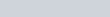## Proof that an expression is divisible by a certain integer (power type)

#### Prove the following:

1. 32n – 1 is divisible by 8
or 32n – 1 is a multiple of 8

#### The method of induction:

• Start by proving that it is true for n=1, then assume true for n=k and prove that it is true for n=k+1. If so it must be true for all positive integer values of n.
Mathematical Induction - Divisibility Tests (1) : ExamSolutions - youtube Video

## Proof that an expression is divisible by a certain integer (non-power type)

#### Prove the following:

1.is divisible by 3
Mathematical Induction - Divisibility Tests (2) : ExamSolutions - youtube Video